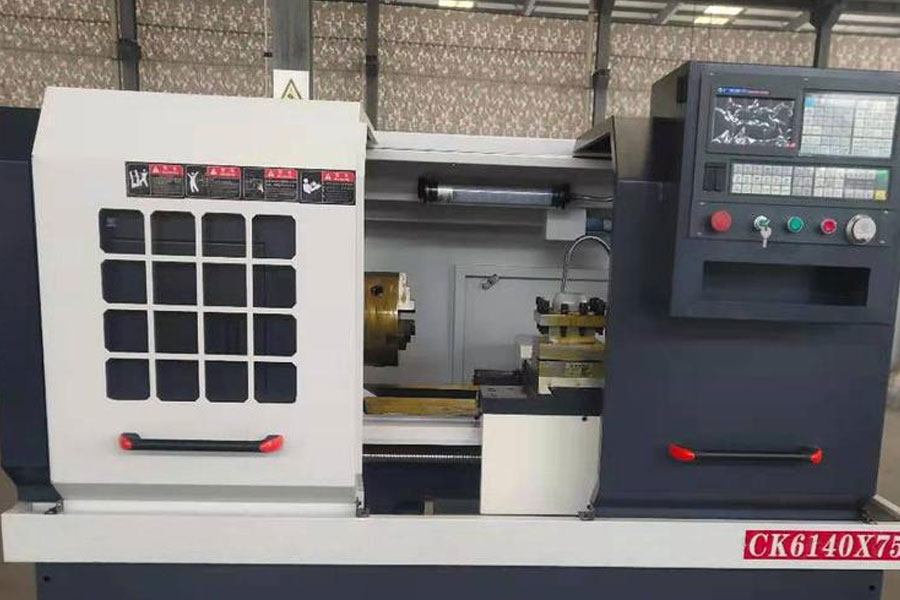## Calculation formula

V=Pi*D*n/1000; it has nothing to do with the feed (F). The specific derivation process is as follows: The so-called linear speed is the relative speed of the contact position of the blade and the workpiece, and has nothing to do with the feed (F). The linear speed is determined according to the tool, workpiece material, and experience. For the lathe, the tool does not move (the feed speed is here) (It can be ignored) The workpiece rotates, so the speed of the contact position between the workpiece and the tool is the cutting linear speed. is: V=Pi*D*n/1000 where Pi is the circumference ratio, D is the rotating diameter of the contact point between the tool and the workpiece, and n is the spindle speed. The unit of  n is revolutions/minute, the unit of D is millimeters, and the unit of the final result V is meters/minute.

## Extended information

Line speed is also divided into average value and instantaneous value. If the time interval taken is very small, the instantaneous linear velocity is obtained in this way. Note that when △t is small enough, the arc AB becomes almost a straight line, and the length of the AB arc is almost the same as the length of the AB line segment. At this time, △l is the displacement of the object from A to B. Therefore, the v here is actually the instantaneous velocity in linear motion, but it is now used to describe circular motion. The linear velocity is a vector with magnitude and direction. For objects that move in a circular motion, its linear velocity changes all the time and always points to the tangent direction of the point.

## related formula

In a uniform circular motion, the linear velocity is equal to the arc length (S) that the moving particle passes and the time (△t) it takes to pass this arc length. That is, v=S/△t, and also v=2πr/T. In a uniform circular motion, although the linear velocity does not change, its direction changes all the time. The relationship between it and angular velocity is v=ω*r. V=ωr=2πrf=2πnr=2πr/T When a moving particle makes a circular motion while also doing another kind of translation, such as a certain point on a car wheel, the linear velocity of the particle at this time is the linear velocity of the circular motion (w*r) and the translational motion. The vector sum of velocity (v): v=w*r+v.